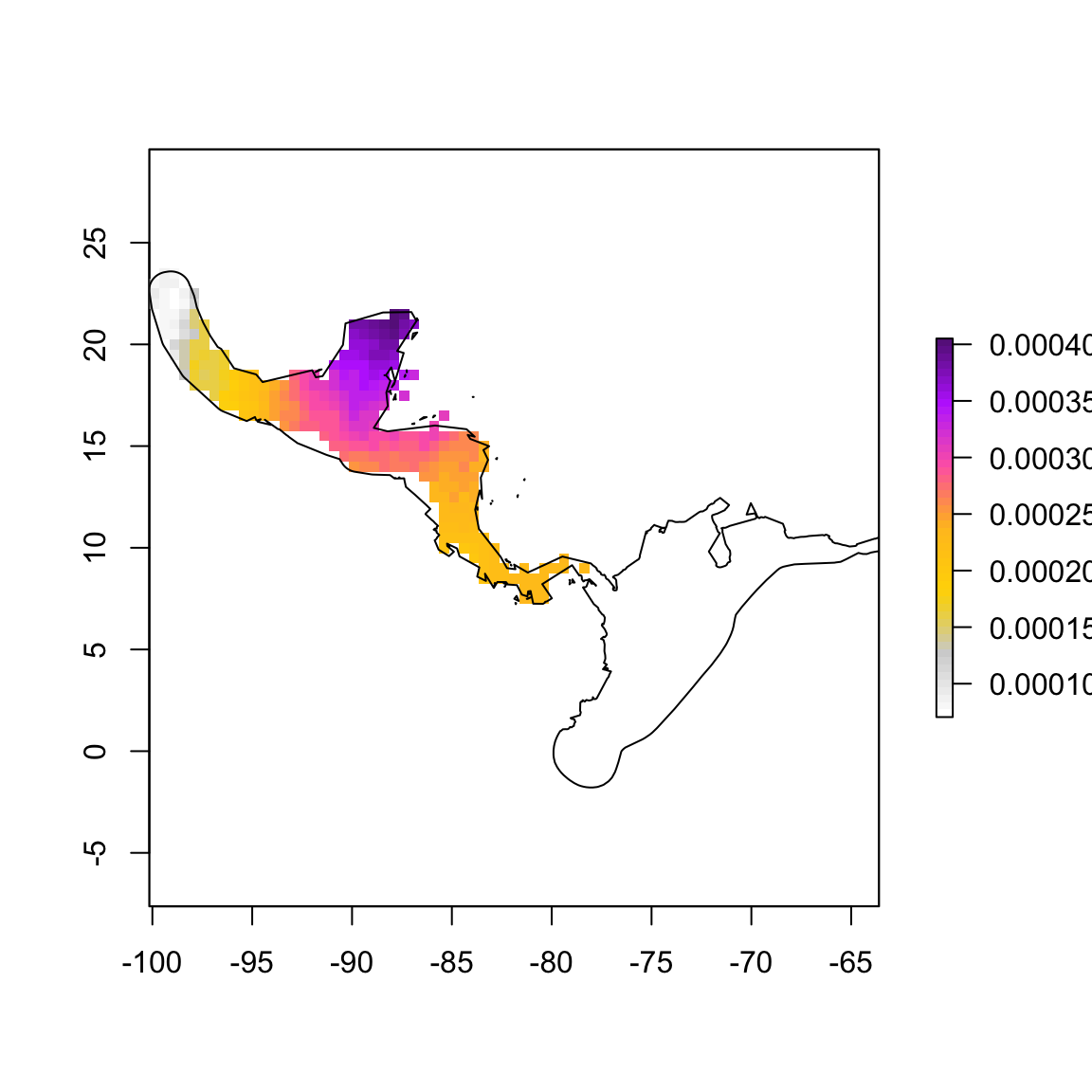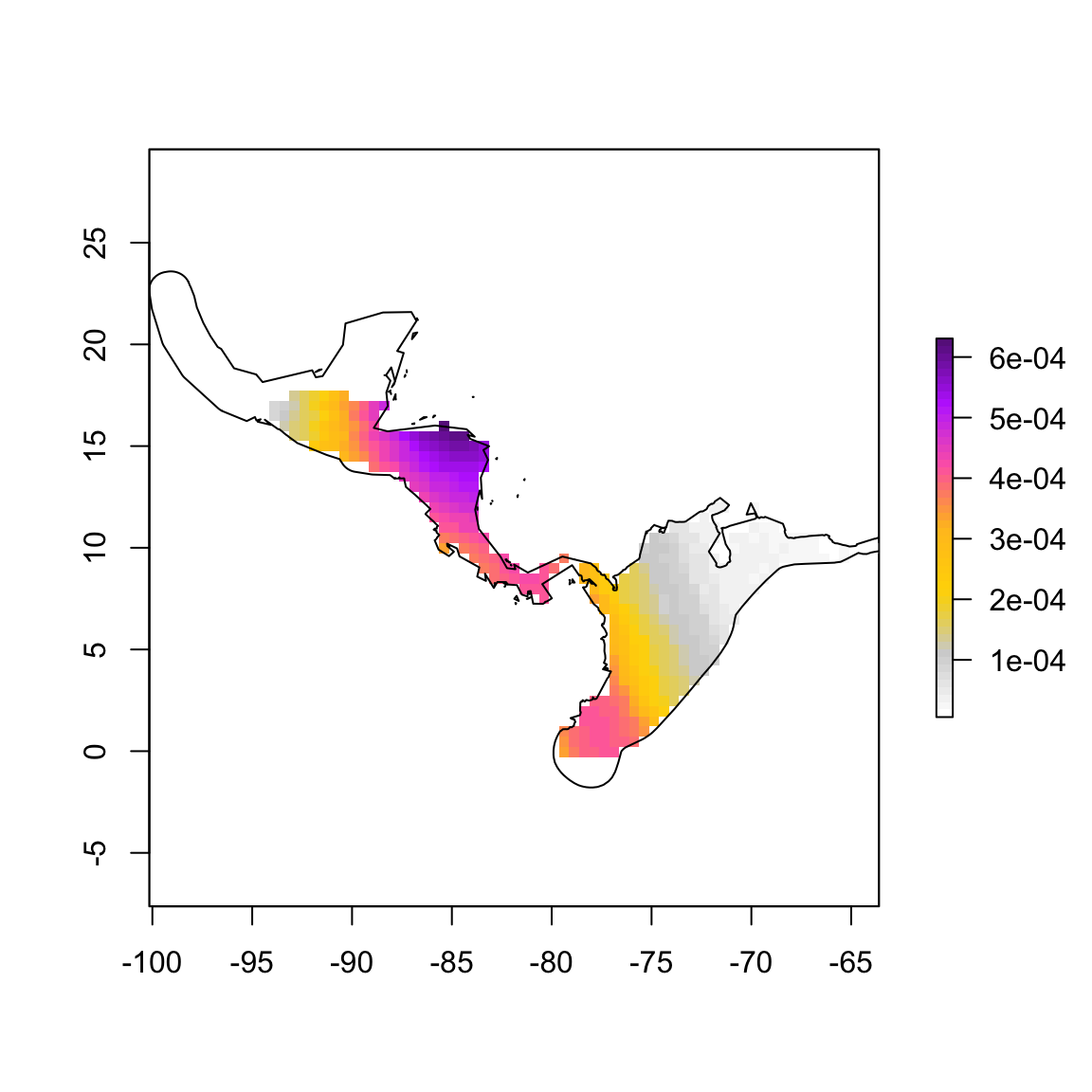``````require(rgdal)
require(rgeos)
require(sp)
require(ggplot2)
require(raster)
library(maps)
library(maptools)
require(devtools)``````

Make some color ramps:

``````cramp1<-colorRampPalette(c("white","lightgray","gold","goldenrod1","hotpink","darkorchid1","darkorchid4"))
bluePH<-rgb(18,127,237, alpha=c(0,255), maxColorValue = 255)
orangeAP<-rgb(255,168,0, alpha=c(0,255), maxColorValue = 255)
redGL<-rgb(232,0,0, alpha=c(0,255), maxColorValue = 255)``````
``````General overview:

1. import nonbreeding rasters
2. replace NAs with 0s and perform PDFs
3. Add populations together, perform PDF
4. Export resulting raster as GeoTiff
5. Plot ``````

Load all .tiff files of the nonbreeding probability density functions of each individual Vermivora from working directory and rasterize:

``````rlist=list.files(getwd(), pattern="tif\$", full.names=FALSE)
for(i in rlist) { assign(unlist(strsplit(i,"[.]")), raster(i)) }``````

Load shapefiles and plot to check:

``````data(wrld_simpl)

``````## OGR data source with driver: ESRI Shapefile
## Source: ".", layer: "gwNB"
## with 11 features
## It has 25 fields
## Integer64 fields read as strings:  FID_NBchry FID_Whemis POP2005``````
``bwNB<-readOGR(dsn=".","bwNB")``
``````## OGR data source with driver: ESRI Shapefile
## Source: ".", layer: "bwNB"
## with 9 features
## It has 14 fields
## Integer64 fields read as strings:  FID_bw_NB_ FID_Whemis POP2005``````
``VermNB<-readOGR(dsn=".","vermNB_D")``
``````## OGR data source with driver: ESRI Shapefile
## Source: ".", layer: "vermNB_D"
## with 1 features
## It has 1 fields``````

Plot an example PDF (ILB04) and overlay nonbreeding distribution of Vermivora:

``````plot(ILB04_ext, col=cramp1(50))Check to ensure surface adds to 1 (i.e., probability density function)

``cellStats(ILB04_ext, sum)``
``##  0.07610879``

Try plotting DMG03:

``````plot(DMG03_ext, col=cramp1(50))Check sum DMG03’s surface:

``cellStats(DMG03_ext, sum)``
``##  0.1131047``

We need to determine the relative probability of the individual warbler being present at one of these cells during the nonbreeding period. To do that, we’ll calculate a probability density function:

First, fix extent of MIB05:

``````MIB05_ext<-projectRaster(MIB05_ext, MIB02_ext)
extent(MIB05_ext)``````
``````## class       : Extent
## xmin        : -100.1573
## xmax        : -63.60464
## ymin        : -1.786311
## ymax        : 23.75048``````
``MIB05_ext``
``````## class       : RasterLayer
## dimensions  : 51, 73, 3723  (nrow, ncol, ncell)
## resolution  : 0.5007215, 0.5007215  (x, y)
## extent      : -100.1573, -63.60464, -1.786311, 23.75048  (xmin, xmax, ymin, ymax)
## coord. ref. : +proj=longlat +datum=WGS84 +no_defs +ellps=WGS84 +towgs84=0,0,0
## data source : in memory
## names       : MIB05_ext
## values      : 0.0001826691, 0.005590735  (min, max)``````
``plot(MIB05_ext, col=cramp1(50))``